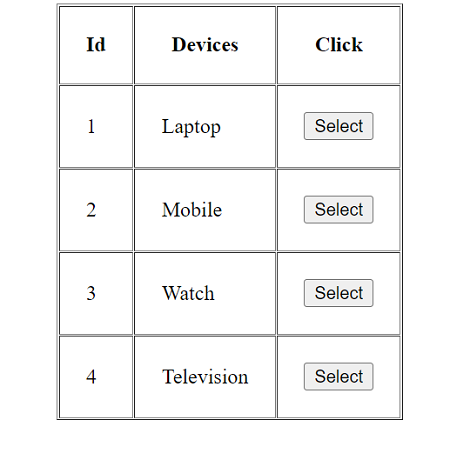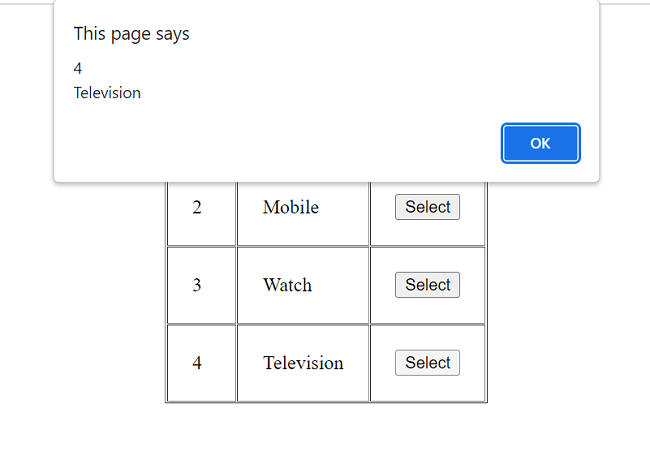# How to get a table cell value using jQuery?

In this tutorial, let's see how can we get the value of a table cell using jQuery?
Submitted by Pratishtha Saxena, on June 26, 2022

Suppose an HTML table is created with some rows and columns in it. So to get the value of the cell we need to first select the row in which that particular cell is present.

For selecting the row in this case, we'll use the closest() method. This returns the first ancestor of the selected element. Also, it begins with the current element of the selected element and goes upward to its ancestors in DOM elements. This will help us here to select the current row.

Syntax:

```\$(this).closest("element");
```

Then after selecting the current row, we'll use the find() method which helps to find all the elements that match the specified selector in the bracket.

Syntax:

```data.find(selector);
```

Then to find the value of different cells in a row, we'll have to specify the cell which will be done using the eq() method in jQuery. This method returns the value with a specific index number of the selected elements. The index numbers here start at 0.

Syntax:

```\$(selector).eq(index);
```

Let's combine all these in an example for better understanding.

HTML Code:

```<html>
<body>
<center>
<tr>
<th>Id</th>
<th>Devices</th>
<th>Click</th>
</tr>
<tr>
<td>1</td>
<td>Laptop</td>
<td><button class="btnSelect">Select</button></td>
</tr>
<tr>
<td>2</td>
<td>Mobile</td>
<td><button class="btnSelect">Select</button></td>
</tr>
<tr>
<td>3</td>
<td>Watch</td>
<td><button class="btnSelect">Select</button></td>
</tr>
<tr>
<td>4</td>
<td>Television</td>
<td><button class="btnSelect">Select</button></td>
</tr>
</table>
</center>
</body>
</html>
```

jQuery Function:

```<script>
\$(".btnSelect").on('click',function(){
var currentRow=\$(this).closest("tr");

var col1=currentRow.find("td:eq(0)").html();
var col2=currentRow.find("td:eq(1)").html();

var data=col1+"\n"+col2;
});
});
</script>
```

Output: